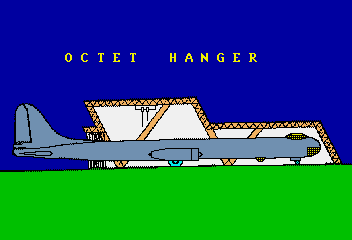# Octet Truss(or time/size-realized isotropic vector matrix)Background Information

Viewed mathematically, as a generic pattern, its hubs correspond to the centers of spheres of equal radius, packed tightly together.

In crystollography, we call this the face-centered cubic lattice (fcc), as distinct from others such as the body-centered cubic (bcc), simple cubic (scp) and hexagonal closest packing (hcp).

Given the uniform distribution of points in such a lattice, plus the equal distances between each point and its 12 nearest neighbors, Fuller called this the isotropic vector matrix (IVM) used it to think about situations with everywhere-the-same energy conditions (what "isotropic" means).

The cctet truss is simply the IVM realized as an artifact for use in engineering applications. These applications range in size from the nanotechnological to the mega-structural -- or any scale in between (see Angle vs Frequency).#### Follow-up Resources

Synergetics on the Web
maintained by Kirby Urner